# Transposed matrix

(diff) ← Older revision | Latest revision (diff) | Newer revision → (diff)
Jump to: navigation, search

The matrix obtained from a given (rectangular or square) matrix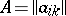(;) by interchanging the rows and the columns, that is, the matrix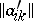, where(;). The number of rows of the transposed matrix is equal to the number of columns of, while the number of columns is equal to the number of rows of. The transpose of a matrixis usually denoted by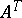or.

#### Comments

Some elementary properties of the transposition of matrices are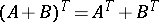,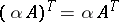,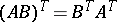,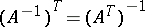.